How Cheenta works to ensure student success?
Explore the Back-Story

# Test of Mathematics Solution Subjective 144 - Finding a Function's Upper BoundThis is a Test of Mathematics Solution Subjective 144 (from ISI Entrance). The book, Test of Mathematics at 10+2 Level is Published by East West Press. This problem book is indispensable for the preparation of I.S.I. B.Stat and B.Math Entrance.

Also visit: I.S.I. & C.M.I. Entrance Course of Cheenta

## Problem

Suppose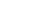is a real valued differentiable function defined on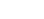with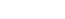. Suppose moreoversatisfies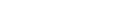Show that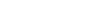for every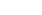## Solution

As the question doesn't require us to find an exact solution rather just an upper bound, we can easily find it by manipulating the given statement after establishing certain properties of.

We see that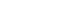for all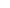which meansis an increasing function.

As the domain iswe can say that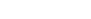for all.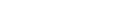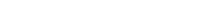(as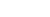)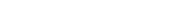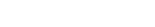Integrating both sides from 1 to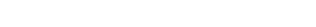Aswe have,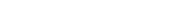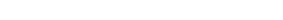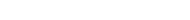Substituting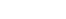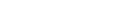Hence Proved.

## Important Resources:This is a Test of Mathematics Solution Subjective 144 (from ISI Entrance). The book, Test of Mathematics at 10+2 Level is Published by East West Press. This problem book is indispensable for the preparation of I.S.I. B.Stat and B.Math Entrance.

Also visit: I.S.I. & C.M.I. Entrance Course of Cheenta

## Problem

Supposeis a real valued differentiable function defined onwith. Suppose moreoversatisfiesShow thatfor every## Solution

As the question doesn't require us to find an exact solution rather just an upper bound, we can easily find it by manipulating the given statement after establishing certain properties of.

We see thatfor allwhich meansis an increasing function.

As the domain iswe can say thatfor all.(as)Integrating both sides from 1 toAswe have,SubstitutingHence Proved.

## Important Resources:

### Knowledge Partner